## Plots

### Logistic Function

$y = \frac{e^{x}}{1+ e^{x}}$

plot.function(function(x){exp(x)/(1+exp(x))},-10,10,ylab="Logistic")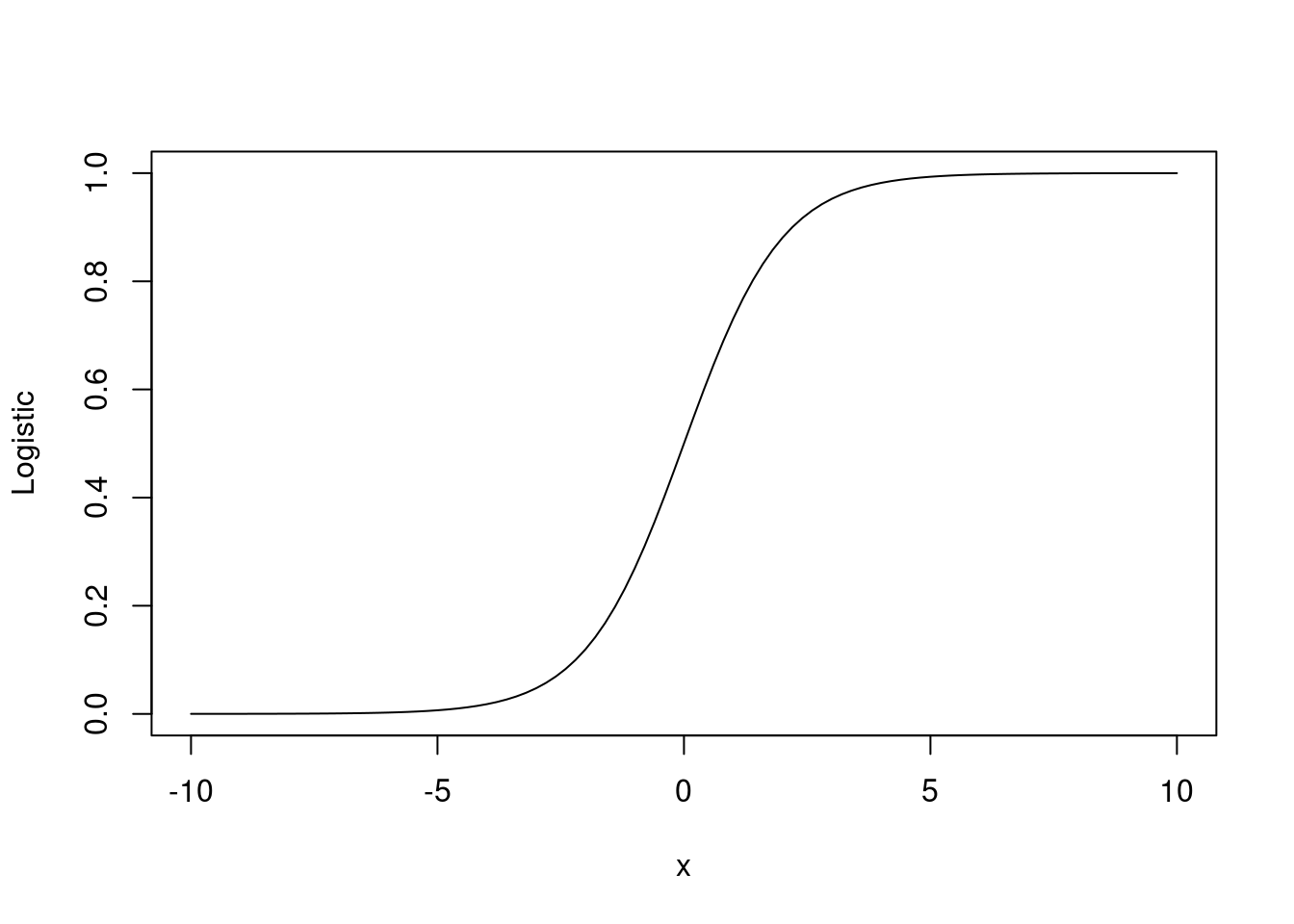### Logit Function

plot.function(function(p){log(p/(1-p))},0,1,ylab="Logit", xlab='p(x)')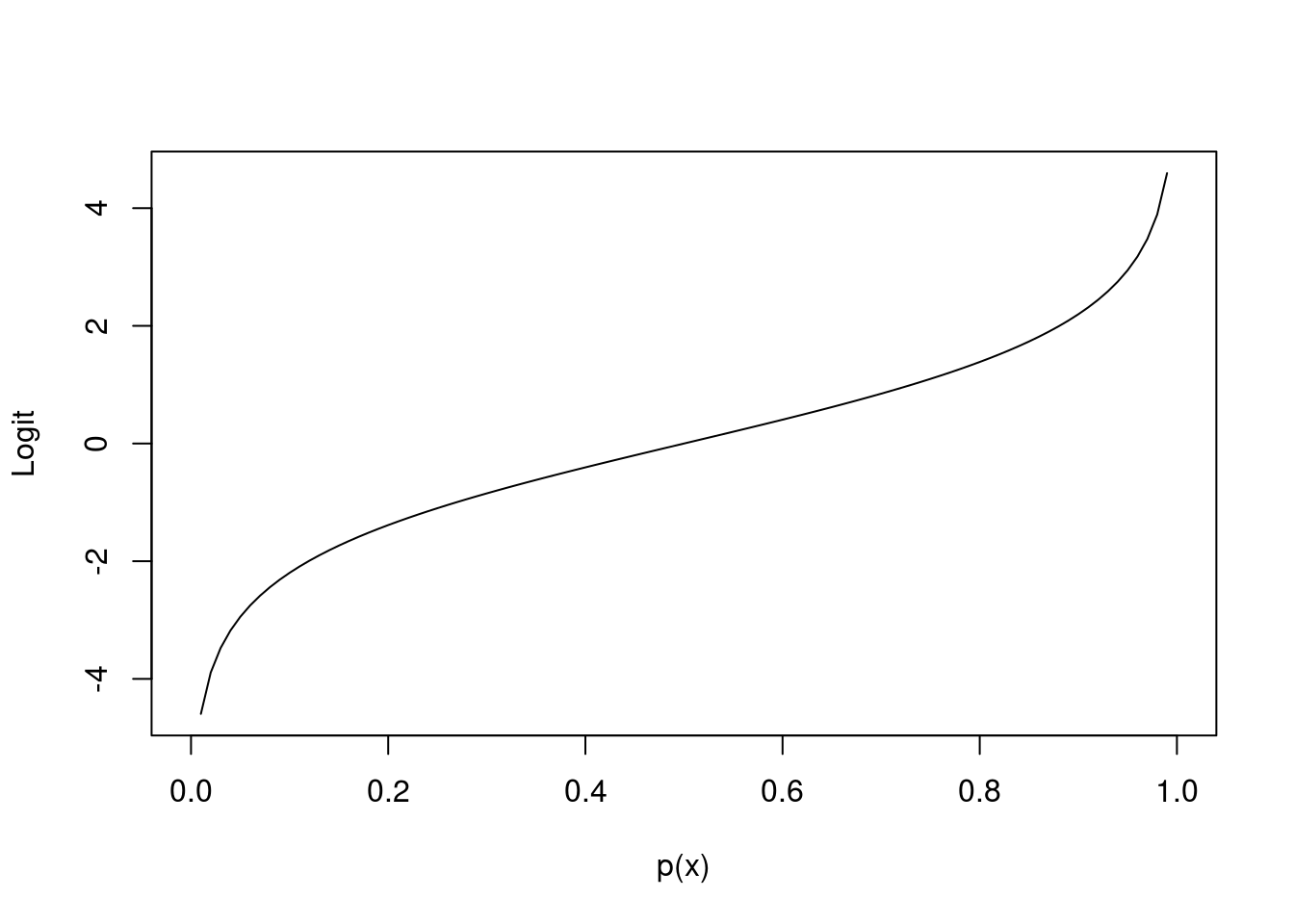## Log Likelihood

Plot log likelihood for estimating the probability of a (possibly biased) coin landing heads. The data is a number of heads h and the number of tails t. The likelihood function is: $\mathcal{L}(p) = p^h \, (1-p)^t ~.$ Some example of a likelihood functions:

#pdf("/tmp/likelihood1.pdf",7,5)
h <- 1; t <- 1
plot.function(function(p){p^h * (1-p)^t},0,1,ylab = "Likelihood", xlab = "p")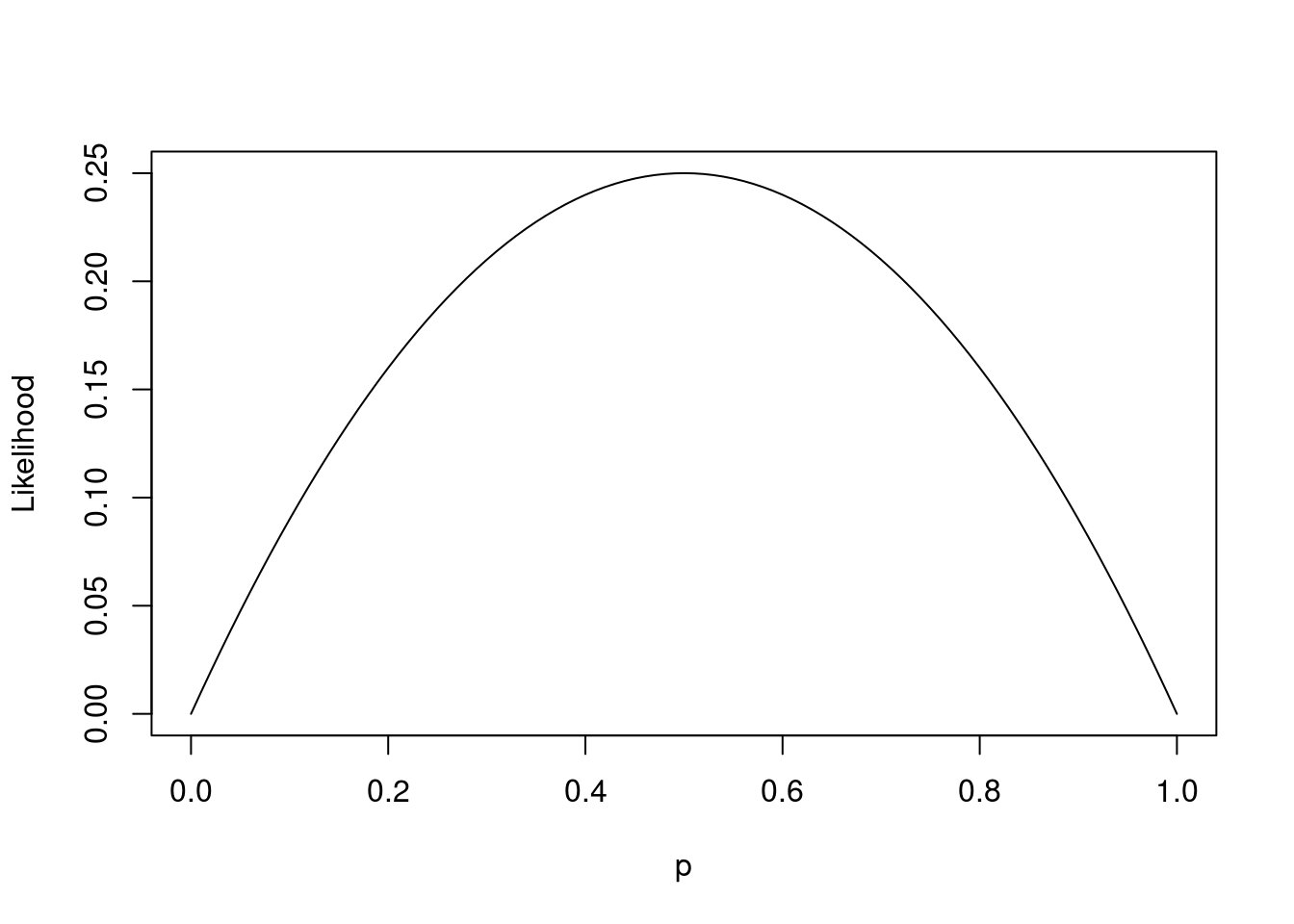#dev.off()
#pdf("/tmp/likelihood10.pdf",7,5)
h <- 10; t <- 10
plot.function(function(p){p^h * (1-p)^t},0,1,ylab = "Likelihood", xlab = "p")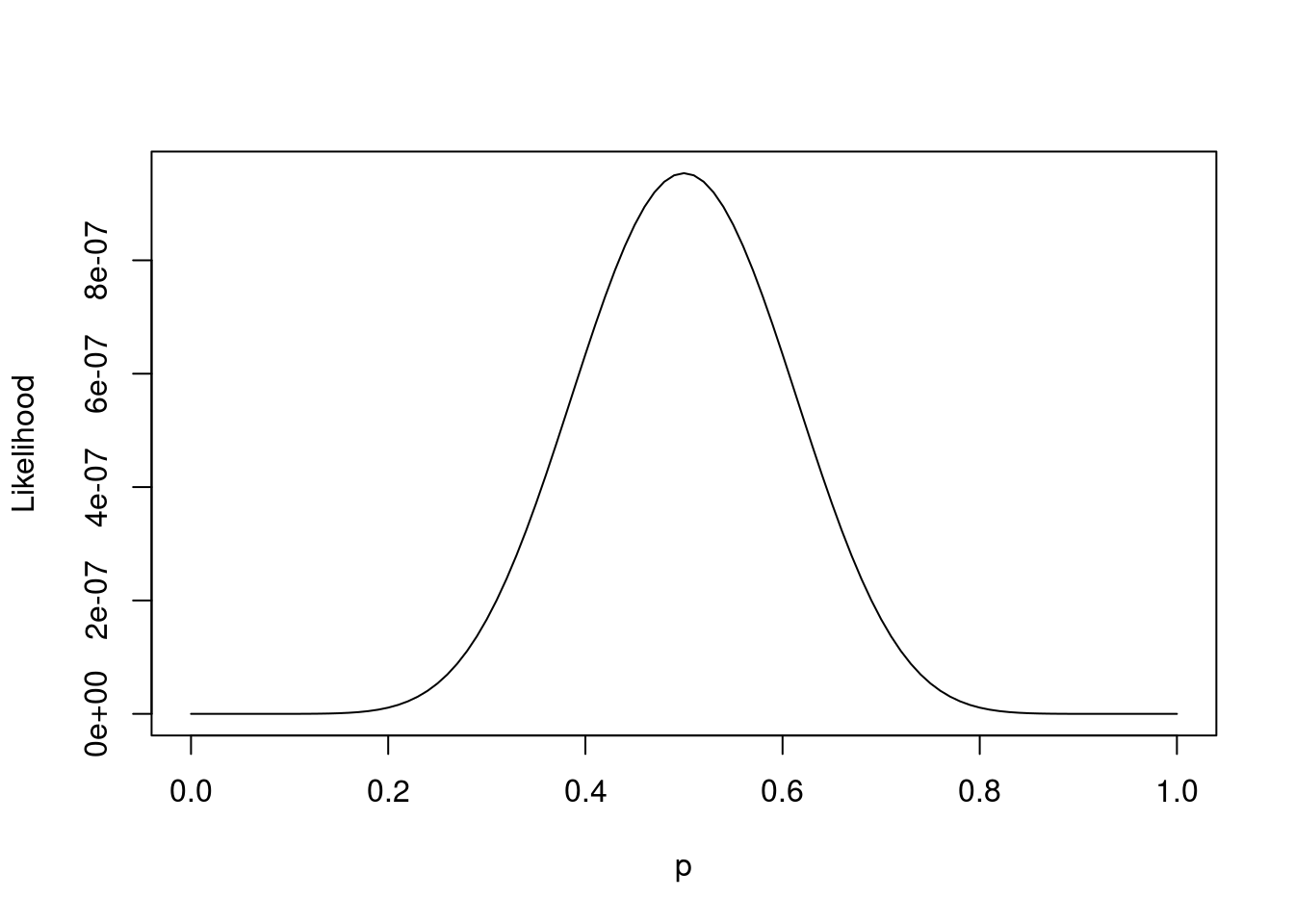#dev.off()
#pdf("/tmp/likelihood100.pdf",7,5)
h <- 100; t <- 100
plot.function(function(p){p^h * (1-p)^t},0,1,ylab = "Likelihood", xlab = "p")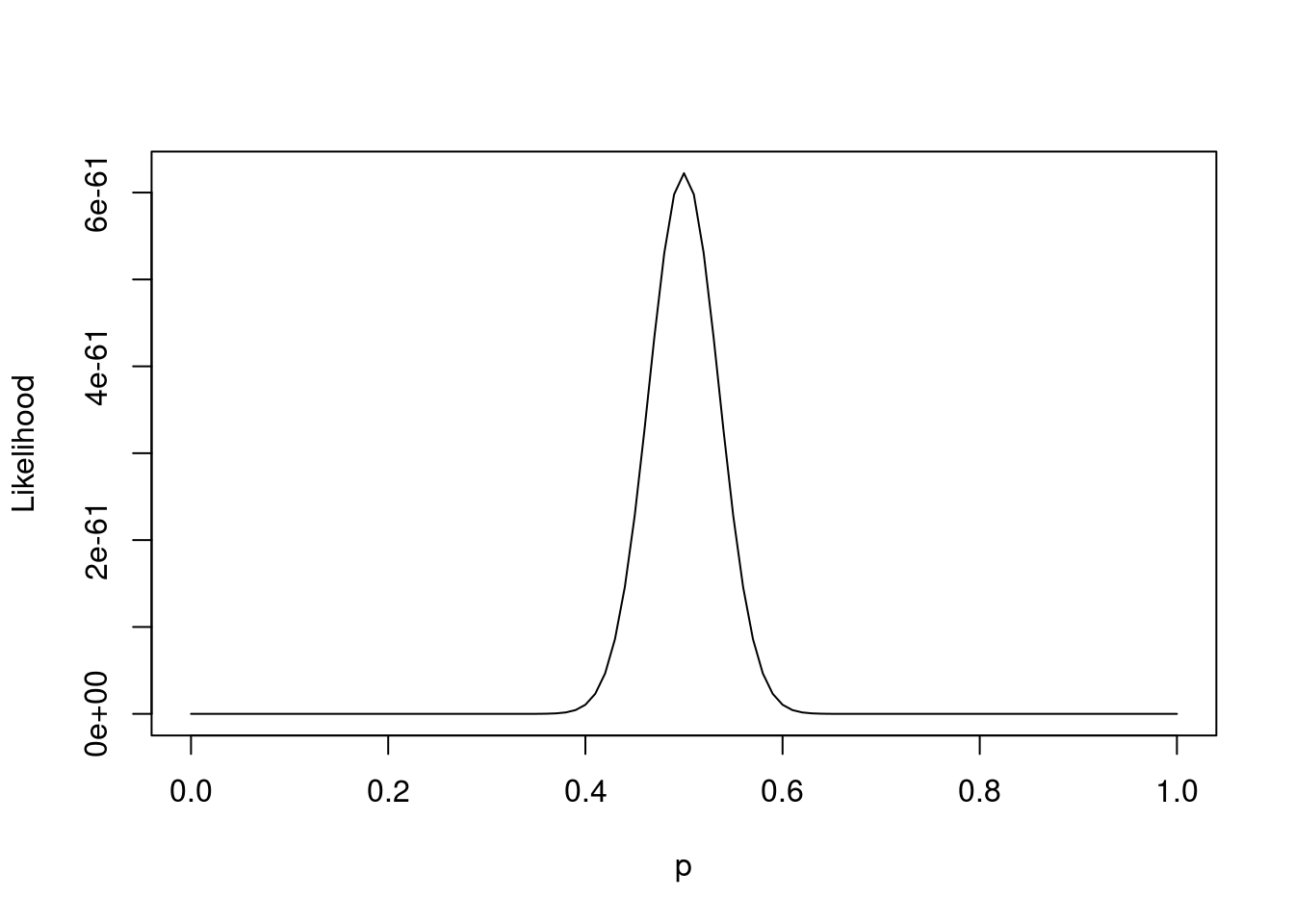#dev.off()

The likelihood function $$\mathcal{L}$$ function is usually difficult to maximize. The function is not concave and the derivative is complex. Instead, we can maximize Log-likelihood $$\log\mathcal{L}$$ which, in this case, is concave. The maximum of a log-likelihood is the same as the maximum of likleihood: $\arg\max_{p} \mathcal{L}(p) = \arg\max_{p} \log\mathcal{L}(p)$ This is because $$\log$$ is a strictly increasing function and the transformation using such function does not change the maximum value.

The plot of the log-likelihood function for an observation with the same number of heads and tails is as follows.

#pdf("/tmp/loglikelihood.pdf",7,5)
h <- 10; t <- 10
plot.function(function(p){h*log(p) + t*log(1-p)},0,1,ylab = "Loglikelihood", xlab = "p")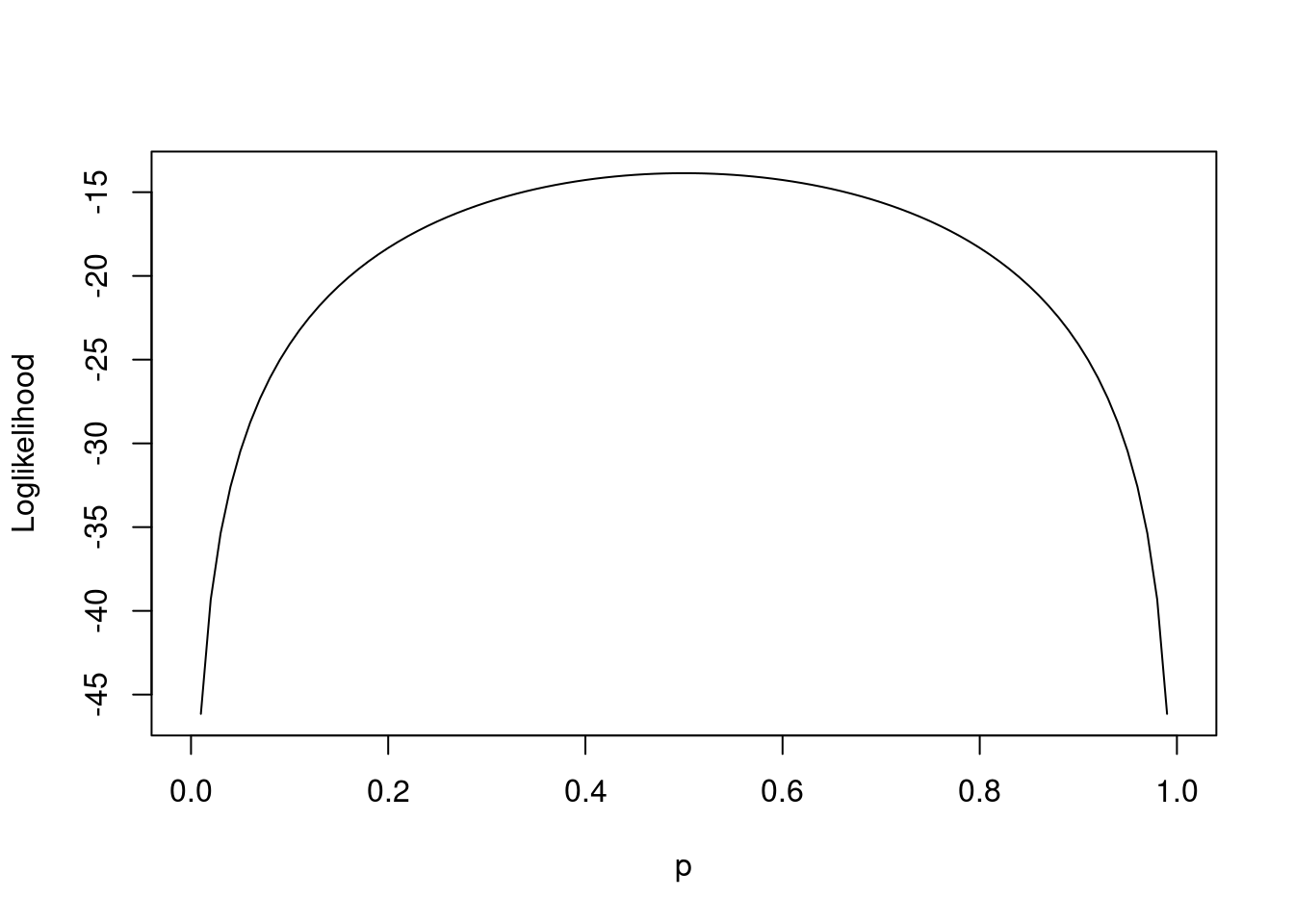#dev.off()

With mode heads than tails, the value of $$p$$ that maximizes the likelihoods becomes biased towards heads.

#pdf("/tmp/loglikelihood_biased.pdf",7,5)
h <- 20; t <- 5
plot.function(function(p){h*log(p) + t*log(1-p)},0,1,ylab = "Loglikelihood", xlab = "p")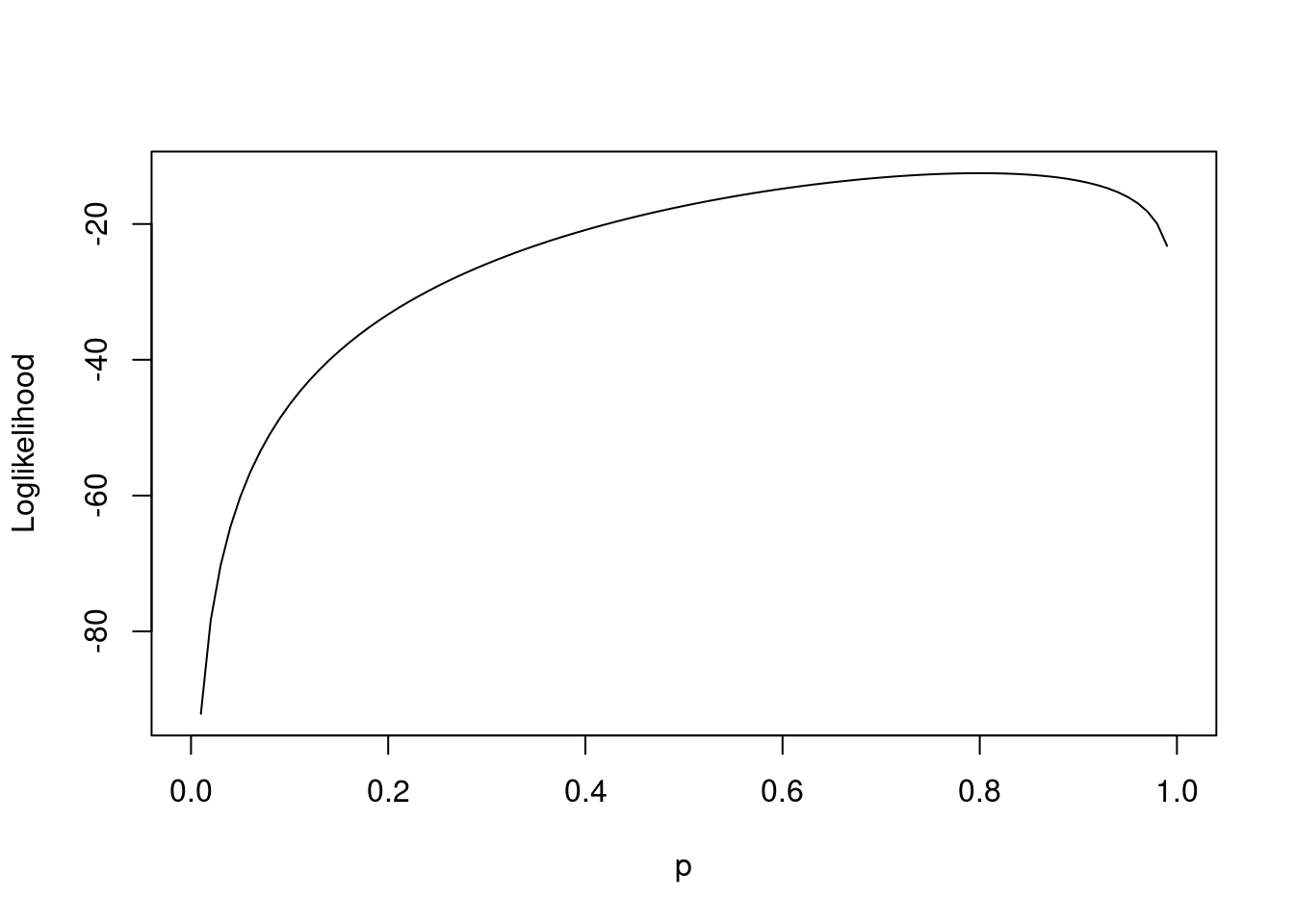#dev.off()